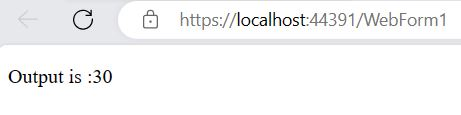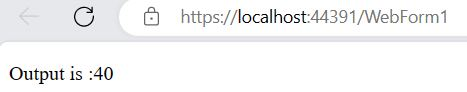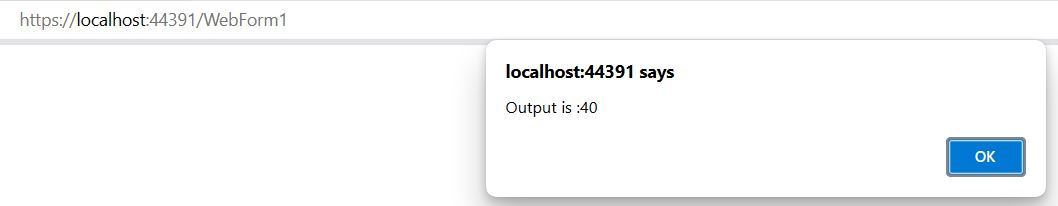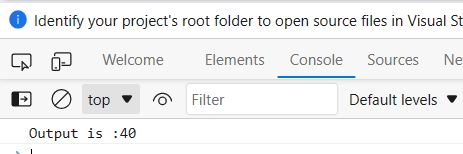﻿ JavaScript Output - TutorialsTrend
This Angular tutorial helps you get started with Angular quickly and effectively through many practical examples.
`

# JavaScript Output

#### JavaScript Generating Output

In JavaScript, There are several different ways to generating output from your JavaScript code.

JavaScript can display data in different ways.

1. innerHTML
2. document.write()
4. console.log()

#### 1. Output Inside an HTML Element

You may also use the innerHTML attribute of an HTML element to write output inside it. However, before we can write the output, we must first pick the element using a method such as getElementById(), as seen in the following example.

Example

``````<!DOCTYPE html>
<html lang="en">
<meta charset="utf-8">
<title>Writing into an HTML Element with JavaScript</title>
<body>
<p id="resultid"></p>
<script>
var x = 5;
var y = 25;
var sum = x + y;
document.getElementById("resultid").innerHTML = "Output is :"+ sum;
</script>
</body>
</html> ``````

Output#### 2. Output with document.write()

You can use the document.write() method to write the content to the current document only while that document is being parsed.

Example

``````<!DOCTYPE html>
<html lang="en">
<meta charset="utf-8">
<title>document.write with JavaScript</title>
<body>
<p id="resultid"></p>
<script>
var x = 15;
var y = 25;
var sum = x + y;
document.write("Output is :" + sum);
</script>
</body>
</html>``````

OutputYou can also use alert dialog boxes to display the message or output data to the user. An alert dialog box is created using the alert() method.

Example

``````<!DOCTYPE html>
<html lang="en">

<meta charset="utf-8">

<body>
<p id="resultid"></p>
<script>
var x = 15;
var y =
25;
var sum = x + y;
</script>
</body>
</html>``````

Output#### 4. Output with console.log()

You can display a message or writes data to the browser console using the console.log() method.

Example

``````<!DOCTYPE html>
<html lang="en">

<meta charset="utf-8">

<body>
<p id="resultid"></p>
<script>
var x = 15;
var y =
25;
var sum = x + y;
console.log("Output is :" + sum);
</script>

</body>
</html>``````

OutputPrev Next